[Introduction]  [Signal arithmetic]  [Signals and noise]   [Smoothing]   [Differentiation]  [Peak Sharpening]  [Harmonic analysis]   [Fourier convolution]  [Fourier deconvolution]  [Fourier filter]  [Wavelets]   [Peak area measurement]  [Linear Least Squares]  [Multicomponent Spectroscopy]  [Iterative Curve Fitting]  [Hyperlinear quantitative absorption spectrophotometry] [Appendix and Case Studies]  [Peak Finding and Measurement]  [iPeak]   [iSignal]  [Peak Fitters]   [iFilter]  [iPower]  [List of downloadable software]  [Interactive tools]

###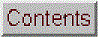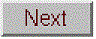Appendix Y: Real-time signal processing (added February 2018)
All of the signal processing techniques covered so far make the assumption that you have acquired and stored the data in computer memory before beginning processing. In some cases, however, it is necessary to do the signal processing in "real time", that is, point-by-point as the data are acquired from the sensor or instrument. That requires some modification of the software, but the main ideas still apply. In this section we will look at ways to perform real-time data plotting, smoothing, differentiation, peak detection, harmonic analysis (frequency spectra), and Fourier filtering. Because the details of the data acquisition itself varies with each individual experimenter and instrumental setup, these demonstration scripts will simulate real-time data so that you can run them immediately on your computer without additional hardware. I'll do this in either of two ways:
(a) by using mouse-clicks to generate each data point, using Matlab's "ginput" function, or
(b) by pre-calculating simulated data and then accessing it point-by-point in a loop.
The first method is illustrated by the simple script realtime.m. When you run this script, it displays a graphical coordinate system; position your mouse pointer along the y (vertical) axis and click to enter data points as you move the mouse pointer up and down. The "ginput" function waits for each click of the mouse button, then the program records the y coordinate position and counts the number of clicks. Data points are assigned to the vector y (line 17), plotted on the graph as black points (line 18), and print out in the command window (line 19). The script realtimeplotautoscale.m is an expanded version that changes the graph scale as the data come in. If the number of data points exceeds 20 ('maxdisplay'), the x axis maximum is  re-scaled to twice that (line 32). If the data amplitude equals or exceeds ('maxy'), the y axis is re-scaled to 1.1 times the data amplitude (line 36).The script realtimeplotautoscale2.m uses the second method to simulate real-time data, using pre-calculated data (loaded from disk in line 13) that is accessed point-by-point in lines 25 and 26 (animation shown on the right).

The script realtimeplotdatedtime.m demonstrates one way to use Matlab's 'clock' function to record the data and time of each data point that is acquired by clicking. You could also have the computer control the time of data acquisition by reading the clock in a loop until the desired time and date arrives, then take a data point. Of course, a Windows machine is not ideal for high-speed, precisely-timed data acquisition, because there are typically so many interrupts and other processes going on in the background, but it's adequate for low-speed applications. For higher speeds, specialized hardware and software is available.

Smoothing. The script RealTimeSmoothTest.m demonstrates real-time smoothing, plotting the raw unsmoothed data as a black line and the smoothed data in red. In this case the script pre-calculates simulated data in line 28 and then accesses the data point-by-point in the processing 'for' loop (lines 30-51). The total number of data points is controlled by 'maxx' in line 17 (initially set to 1000) and the smooth width (in points) is controlled by 'SmoothWidth' in line 20. (To do this with real time data from your sensor, comment out line 29 and replace line 32 with the code that acquires one data point from your sensor).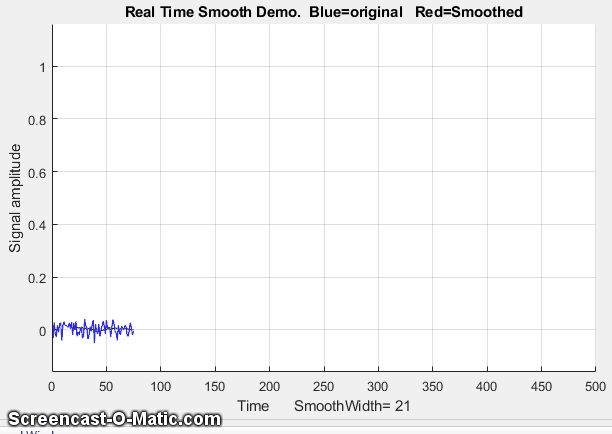As you can see in the screen animation on the left, the smoothed data (in red) is delayed compared to the raw data, because a smoothed data point can not be computed until a number of data points equal to the smooth width has been acquired - 21 points, or about 0.5 seconds in this example. (However, knowing the smooth width, you can correct the recorded y-axis positions of signal features, such as maxima, minima, peaks, or inflection points). This particular example implements a sliding average smooth, but other smooth shapes can be implemented simply by uncommenting line 24 (rectangular smooth), 25 (triangular smooth), or 26 (Gaussian smooth), which requires that the functions 'triangle' and 'gaussian' be in the Matlab/Octave path.

A practical application of a sliding average smooth like this is in a control system where a noisy signal turns on a valve, switch or alarm signal whenever the signal exceeds a certain value; an example is shown in the figure on the right,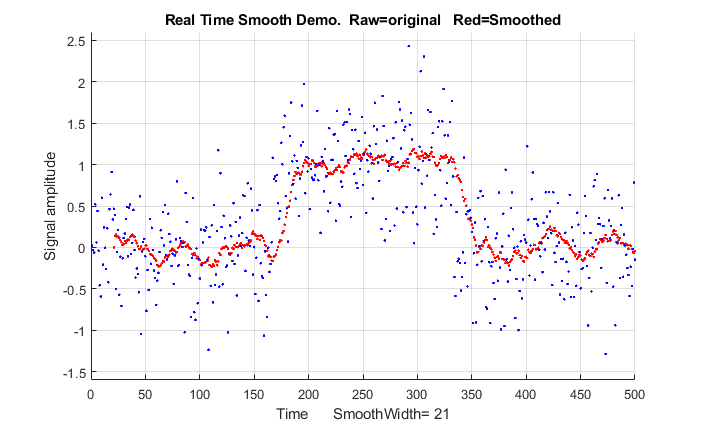where the threshold value is 0.5 and the signal is so noisy that smoothing is required to prevent the signal from prematurely triggering the control. Too much smoothing, however, will cause an unacceptable delay in operation.

On a standard desktop PC (Intel Core i5 3 Ghz) running Windows 10 home, the smooth operation adds about
2 microseconds per data point to the data acquisition time (without plotting, PlottingOn=0) and 20 milliseconds per point (50 Hz) with point-by-point plotting (PlottingOn=1). With plotting off, the script acquires, smooths, and stores the smoothed data in the variable "sy" in real time, then plots the data only after data acquisition is complete, which is much faster than plotting in real time.

Differentiation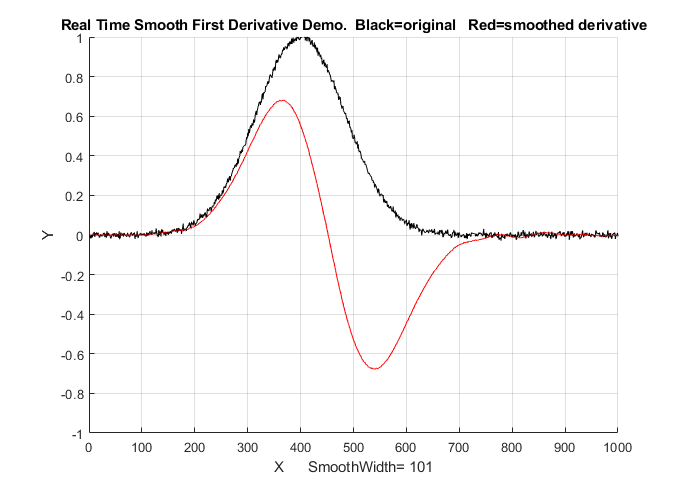. The script RealTimeSmoothFirstDerivative.m demonstrates real-time smoothed differentiation, using a simple adjacent-difference algorithm (line 47) and plotting the raw data as a black line and the first derivative data in red. The demonstration script RealTimeSmoothSecondDerivative.m
computes the smoothed second derivative by using a central difference algorithm (line 47). Both of these scripts pre-calculate the simulated data in line 28 and then accesses the data point-by-point in the processing loop (lines 31-52).  In both cases the maximum number of points is set in line 17 and the smooth width is set in line 20. Again, the derivatives are delayed compared to the original signal. Any derivative order can be calculated this way using the derivative coefficients in the Matlab/Octave derivative functions listed on Differentiation.html#Matlab.

Peak detection. The little script realtimepeak.m demonstrates simple real-time peak detection based on derivative zero-crossing, using mouse clicks to simulate data. Each time your mouse clicks form a peak (that is, go up and then down again), the program will register and label the peak on the graph and print out its n and y values.

Peak detected at n=13 and y=7.836

Peak detected at n=26 and y=1.707

I
n this case, a peak is defined as any data point that has lower amplitude points adjacent to it on both sides, which is determined by the nested 'for' loops in lines 31-36. Of course, a peak can not be registered until the point following the peak is recorded, because there is no way to predict ahead of time whether that point will be lower or higher than the previous point.

If the data are noisy, it's better to smooth the data stream before detecting the peaks, which is exactly what RealTimeSmoothedPeakDetection.m does, which
reduces the chance of false peaks due to random noise but has the disadvantage of delaying the peak detection further. Even better, the script RealTimeSmoothedPeakDetectionGauss.m uses the technique described on PeakFindingandMeasurement.htm#findpeaks; it locates the positiv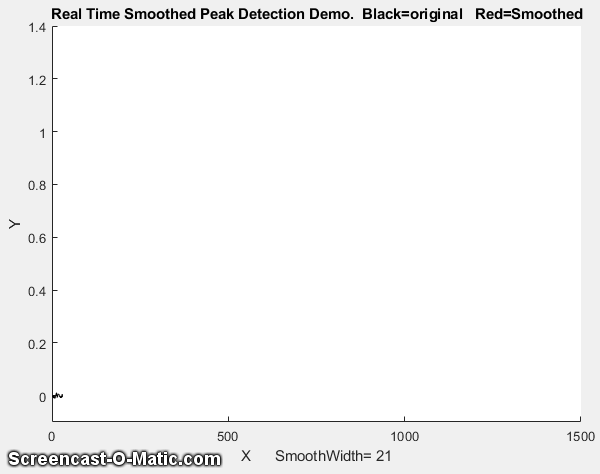e peaks in a noisy data set that rise above a set amplitude threshold ("AmpThreshold" in line 55), performs a least-squares curve-fit of a Gaussian function to the top part of the raw data peak (in line 58), identifies each peak (line 59), computes the position, height, and width (FWHM) of each peak from that least-squares fit, and prints out each peak found in the command window. The peak parameters are measured on the raw data, so they are not distorted by smoothing. If you look at the animation on the right, you can see that the "peak" label appears next to each detected peak just a fraction of a second after the top of the peak, but the actual peak times listed are not delayed. In this example, the actual peak times are x=500, 1000, 1100, 1200, 1400. (Also note that the first visible peak, at x=300, is not detected because it falls below the amplitude threshold, which is 0.1 in this case).

Peak detected at x=500.1705, y=0.42004, width= 61.7559
Peak detected at x=1000.0749, y=0.18477, width= 61.8195
Peak detected at x=1100.033, y=1.2817, width= 60.1692
Peak detected at x=1199.8493, y=0.36407, width= 63.8316
Peak detected at x=1400.1473, y=0.26134, width= 58.9345

The script additionally numbers the peaks and saves the peak parameters of all the peaks in a matrix called PeakTable, which you can interrogate as each peak is encountered if you are looking for particular peak patterns.  See PeakFindingandMeasurement.htm#UsingP for some ideas on using Matlab/Octave notation and functions to do this.

Peak sharpening.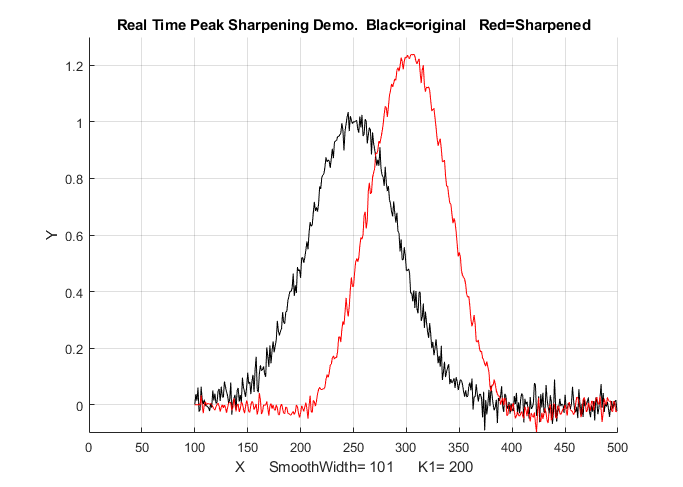The script RealTimePeakSharpening.m demonstrates real-time peak sharpening using the second derivative technique. It uses pre-calculated simulated data in line 30 and then accesses the data point-by-point in the processing loop (lines 33-55). In both cases the maximum number of points is set in line 17, the smooth width is set in line 20, and the weighting factor (K1) is set in line 21. In this example on the left, the smooth width is 101 points, which accounts for the the delay in the sharpened peak compared to the original (about 1.12 seconds without real-time plotting and 3.8 second with real-time plotting).

Real-Time Frequency Spectrum. The script RealTimeFrequencySpectrumWindow.m computes and plots the Fourier frequency spectrum of a signal. Like the scripts above, it loads the simulated real-time data from a ".mat file" and then accesses that data point-by-point in the processing loop. A critical variable in this case is "WindowWidth", the number of data points taken to compute each frequency spectrum. The larger this number, the fewer the number of spectra that will be generated, but the higher will be the frequency resolution. On a standard desktop PC (Intel Core i5 3 Ghz running Windows 10 home), this script generates about 50 spectra per second with an average data rate (points per seconds) of about 50,000 Hz. Smaller spectra (i.e. lower values of WindowWidth) generate proportionally lower average data rates (because the signal stream is interrupted more often to calculate and graph a spectrum).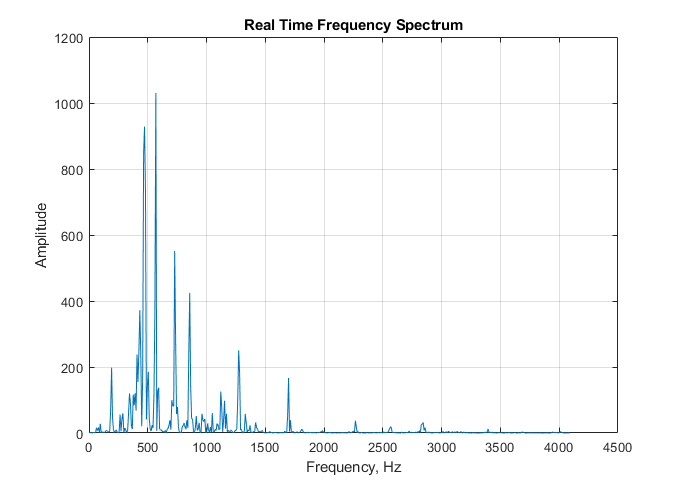If the data stream is an audio signal, it's also possible to play the sound through the computer's sound system synchronized with the display of the frequency spectra; to do this, set PlaySound=1. Each segment of the signal is played, just before the spectrum of that segment is displayed, as shown on the right. The sound reproduction will not be not perfect, because of the slight delay while the computer computes and displays the spectrum before going on to the next segment. In this demonstration script, the data file is in fact an audio recording of an 8-second excerpt of the 'Hallelujah Chorus' from Handel's Messiah with a sampling rate of 8192 Hz, which is included in the Matlab distribution. The figure above shows one of the 70 spectra generated with a WindowWidth of 1024. You can adjust the argument of the 'pause' function for your computer to minimize this problem and to make the sound play at the correct pitch.

Real-Time Fourier Filter. The script RealTimeFourierFilter.m is a demonstration of a real-time Fourier filter. It pre-computes a simulated signal starting in line 38, then access the data point-by-point (line 56, 57), and divides up the data stream into segments to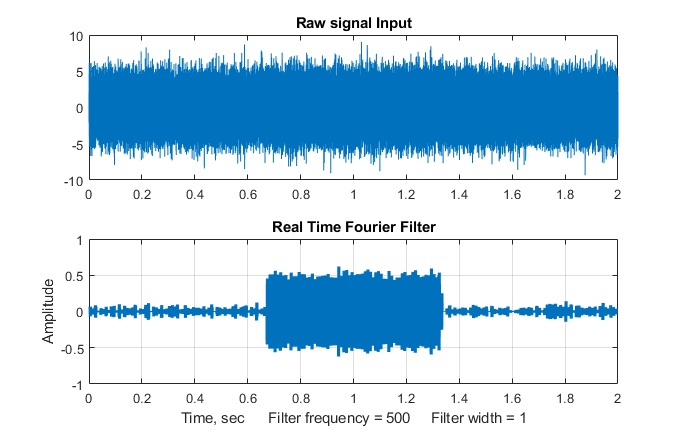compute each filtered section. "WindowWidth" (line 55) is the number of data points in each segment. The larger this number, the fewer the number of segments that will be generated, but the higher will be the frequency resolution within each segment. On a standard desktop PC (Intel Core i5 3 Ghz running Windows 10 home), with a window width of 1000 points, this script generates about 35 filtered segments per second with an average data rate (points per second) of about 34,000 Hz. Smaller segments (i.e. lower values of WindowWidth) generate proportionally lower average data rate (because the signal stream is interrupted more often to calculate and graph the filtered spectrum). The result of applying the filter to each segment is displayed in real time during the data acquisition, and then at the end the script compares the entire raw signal input to the reassembled filtered output, shown the the figure on the left.

In this demonstration, a bandpass filter is used to detect a 500 Hz
sine wave (the frequency is controlled by 'f' in line 28) that occurs in the middle third of a very noisy signal (line 32), from about 0.7 sec to 1.3 sec. The 500 Hz sine wave is so weak it can not be seen at all in the raw signal (upper panel of the figure on the left). The filter center frequency (CenterFrequency) and width (FilterWidth) are set in lines 46 and 47.

Real-time classical least squares. The classical least squares technique can be applied in real time to 2D chromatography with array detectors that can acquire a complete spectrum multiple times per second over the entire chromatogram. This is explored in "Spectroscopy and chromatography combined: time-resolved Classical Least Squares".

To apply any of these examples to real-time data from your sensor or instrument, you need only the main processing 'for' loop, replacing the first lines after the 'for' statement with a call to a function that acquires a single point of raw data and assigns it to y(n). If you don't need the data plotted out point-by-point in real time, you can speed things up greatly by removing the "drawnow" statement at the end of the 'for' loop or by removing all the plotting code.

In the examples here, the output of the processing operation is used to plot or to print out the processed data point-by-point, but of course it could also be used as the input to another processing function or to a digital-to-analog converter or simply to trigger an alarm if certain specified results are obtained (e.g. if the signal exceeds a certain value for a specified length of time, or if a peak is detected at a specified position or height, etc).

This page is part of "A Pragmatic Introduction to Signal Processing", created and maintained by Prof. Tom O'Haver , Department of Chemistry and Biochemistry, The University of Maryland at College Park. Comments, suggestions and questions should be directed to Prof. O'Haver at toh@umd.edu. Updated July, 2022.